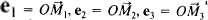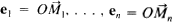# basis

(redirected from basis pedunculi cerebri)
Also found in: Dictionary, Thesaurus, Medical, Legal, Financial.

## basis

Maths (of a vector space) a maximal set of linearly independent vectors, in terms of which all the elements of the space are uniquely expressible, and the number of which is the dimension of the space

## Basis

in mathematics, a set of linearly independent vectors taken in a certain order and plotted from a common origin. For vectors in three-dimensional space, any set of three vectorsthat are not parallel to the same plane can be used as a basis. If the vectors making up the basis are mutually orthogonal, then the basis is said to be orthogonal; if the vectors are of unit length, then the basis is an orthonormal basis. In the general case of an n-dimensional vector space, any set of n linearly independent vectorscan be taken as a basis.

## basis

[′bā·səs]
(mathematics)
A set of linearly independent vectors in a vector space such that each vector in the space is a linear combination of vectors from the set.
Site: Follow: Share:
Open / Close